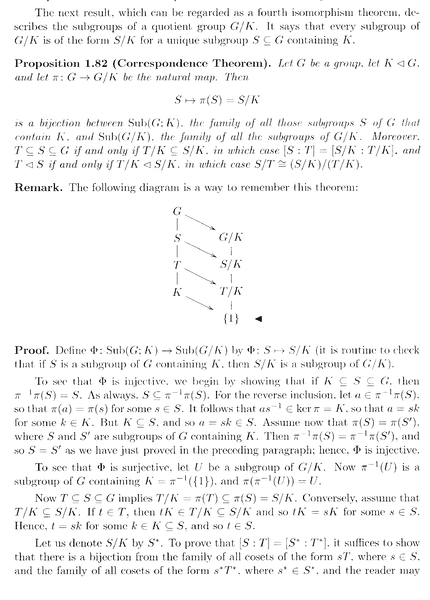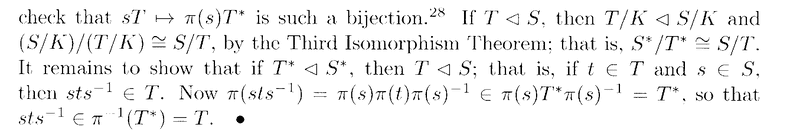# Correspondence Theorem for Groups ... Another Question ...

Gold Member
I am reading the book: "Advanced Modern Algebra" (Second Edition) by Joseph J. Rotman ...

I am currently focused on Chapter 1: Groups I ...

I need help with an aspect of the proof of Proposition 1.82 (Correspondence Theorem) ...

Proposition 1.82 reads as follows:In the above proof by Rotman we read the following:

" ... ... To see that ##\Phi## is surjective, let ##U## be a subgroup of ##G/K##. Now ##\pi^{-1} (U)## is a subgroup of ##G## containing ##K = \pi^{-1} ( \{ 1 \} )##, and ##\pi ( \pi^{-1} (U) ) = U## ... ... "

My questions on the above are as follows:

Question 1

How/why is ##\pi^{-1} (U)## is a subgroup of ##G## containing ##K##? And further, how does ##\pi^{-1} (U) = \pi^{-1} ( \{ 1 \} )## ... ... ?

Question 2

How/why exactly do we get ##\pi ( \pi^{-1} (U) ) = U##? Further, how does this demonstrate that ##\Phi## is surjective?

Help will be much appreciated ...

Peter

## Answers and Replies

member 587159
Hi Peter

Question 1:

The preimage of a subgroup of the codomain is always a subgroup of the domain. Try to prove this using the characterisation of subgroups

(i.e., show that ##\pi^{-1}(U) \neq \emptyset## and ##a,b \in \pi^{-1}(U) \implies ab^{-1} \in \pi^{-1}(U)##)

##K \subseteq \pi^{-1}(U)## because ##K = \pi^{-1}(\{1\}) = \pi^{-1}(\{e_{G/K}\})##

Question 2:

Furthermore, for any surjective function:

##\pi(\pi^{-1}(U)) = U##.

Try to prove this (set theory question, has nothing to do with groups)

This shows that ##\Phi## is surjective, because you took an arbitrary ##U## subgroup of ##G/K## and then show that ##\Phi(\pi^{-1}(U)) = \pi^{-1}(U)/K =\pi(\pi^{-1}(U)) = U##.

Note that it is important here that ##\pi^{-1}(U)## contains ##K##, otherwise the quotient is not well defined.

Gold Member
Thanks Math_QED ... appreciate your help and support...

Just now reflecting on what you have written ...

Peter

Gold Member
Hi Math_QED ...

Taking up your suggestion ...

We have that ##U## is a subgroup of ##G/K## ...

Want to show that ##\pi^{-1} (U) \neq \emptyset## and that ##a,b \in \pi^{-1} (U) \Longrightarrow ab^{-1} \in \pi^{-1} (U)##

Now ...

##\pi( 1_G ) = 1_{ G/K } \Longrightarrow \pi^{-1} ( 1_{ G/K } ) = 1_G##

##\Longrightarrow \pi^{-1} (U) \neq \emptyset##

Now ...

... let ##a, b \in \pi^{-1} (U)##

##\Longrightarrow \pi(a), \pi(b) \in U##

##\Longrightarrow \pi(a), [ \pi(b) ]^{-1} \in U## since ##U## is a subgroup ...

##\Longrightarrow \pi(a), \pi( b^{-1} ) \in U## since ##\pi## is a homomorphism ...

##\Longrightarrow \pi(a) \pi( b^{-1} ) \in U## since ##U## is a subgroup ...

##\Longrightarrow \pi( a b^{-1} ) \in U## since ##\pi## is a homomorphism ...

##\Longrightarrow a b^{-1} \in \pi^{-1} (U)## ...

Therefore ##\pi^{-1} (U)## is a subgroup of ##G## ...

Is that correct ...?

Peter

fresh_42
Mentor
Is that correct ...?
Yes, it is.

•Math Amateur
member 587159
Hi Math_QED ...

##\pi( 1_G ) = 1_{ G/K } \Longrightarrow \pi^{-1} ( 1_{ G/K } ) = 1_G##

##\Longrightarrow \pi^{-1} (U) \neq \emptyset##

Is that correct ...?

Peter

The idea is correct, but the line

##\pi( 1_G ) = 1_{ G/K } \Longrightarrow \pi^{-1} ( 1_{ G/K } ) = 1_G##

doesn't make sense. The preimage is a set, and can't be equal to something that isn't a set. Even if you would have written ##\{1_G\}##, it wouldn't be correct though.

Notice that ##\pi^{-1}(1_{G/K})## is shorthand notation for ##\pi^{-1}(\{1_{G/K}\})##. We are not talking about inverse functions here.

You should rather write ##\pi(1_G) = 1_{G/K} \in U##, which means that ##1_G \in \pi^{-1}(U)##. Can you explain me why ##1_{G/K} \in U##? This is essential here.

The rest is correct.

Last edited by a moderator:
•Math Amateur and fresh_42